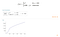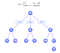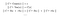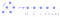TLDR: We developed a library for encoding and evaluating math expression on-chain. Bonding curve demo contract is available Rinkeby testnet. Check it out!

At Band Protocol, we are building a set of tools to enable effective information curation in decentralized communities. One of the challenges we faced while developing the first version of Band Protocol on Ethereum is how to store and evaluate arbitrary mathematical expressions on-chain. In this article, we will go over Band Protocol’s take on solving this problem using on-chain expression trees. The end goal is to have a Solidity library that can store and evaluate arbitrary expressions with single variable (example below) on the blockchain.Example of complex math expression to evaluate on chain with Solidity (generated with Wolfram|Alpha)

Use Cases

• Bonding curve: Bonding Curve token issuance contract could use the library to power its logic. Existing implementations, such as the ones from Bancor and Relevant, only permit exponential reserve-ratio equations. With our library, the set of possible bonding equations expands, and people can start experimenting more things.
• Token vesting schedule: The current goto smart contract for token vesting, implemented by OpenZeppelin, assumes the standard cliff then linear vesting schedule. While this is sufficient in most cases, it does not support common features like monthly vesting. By formulating as a mathematical expression, the contract could support more flexible vesting schedules while still retaining its simplicity.
• TCR deposit: At Band Protocol, we are exploring a variant of Token Curated Registry with independent decay for each entry’s min_deposit constraint. In other words, as an entry lives longer, the required deposit decreases. Equation library can be used to dictate decay rate.

Specification, and Design Goals

With that, we aim for the library the have the following interface containing two functions.

library Equation {
// Abstract data type that encapsulates a mathematical expression
struct Data {
...
}

// Initialize this expression from some TBD kinds of arguments
function init(Data storage self, ... some arguments ...);

// Evaluate this expression with given value 'x' in the expression
function calculate(Data storage self, uint256 x);
}

There are three main design goals that we would like the library to achieve. (Hint: we achieved all of them)

• Simplicity: Running on an immutable ledger with potential high stake, the library should be simple and easy to understand. Ultimately in the future, we would like the evaluation rules and implementations to be formally verifiable and provable correct.
• Extensibility: The representation must be generic and extensible. Adding new building blocks for a new kind of equations should be simple and not disruptive.
• Efficiency/Scalability: Last but not least, since expression evaluations are done on-chain. Gas cost must be reasonable. We expect the library to take less then 10,000 gas for each expression evaluation. That’s lower than storing a single word on the blockchain!

Introducing N-nary Expression Tree

We surely are not the first guys to face this problem of making sense of math expressions. In fact, most compilers and interpreters do it all the time using expression tree! An expression tree is essentially a tree in which each internal node corresponds to an operator, while each leaf node corresponds to a term (operand). The example below shows an expression and its corresponding tree. Node that each internal node can have 1, 2, or 3 children depending on its operator.Example of a function with branching, and its expression tree representation

Evaluation Rules

Evaluation rule is quite intuitive — very similar to how ordinary people do math. Evaluating an expression Eunder a context C (which in this case contains the value of variable x) is done by evaluating all of E’s sub-expressions under C, then combine the results with E’s operator. The following shows denotation semantics of some of the constructs.Formal evaluation rule of some of the opcodes currently available with the library

Parsing a readable math term to an expression tree

Algorithms like Shunting-yard algorithm can be used to process input string into its polish notation. This notation will then be passed to the library’s init function as a pre-order traversal list of opcodes to instantiate the expression in Solidity, with each operator encoded to a unique integer per spec. Constants are preceded with 0opcode. Note that we intentionally abstract the parsing complication mess out of the blockchain to simplify on-chain computation. See example below for parsing x^2 + 10. The process of converting an expression string to opcode list can also be done automatically via an off-chain web application (see future work).Example of conversion from expression tree to the raw value passed to the library

Back to Solidity World and Demo!

The source is currently available at Band Protocol’s smart contracts repository. In this section, we will go over it and explain the important parts of the library.

Representing a Tree On-Chain

A node is represented as a struct containing its opcode as well as the locations of its children or in the case of Constant, its value. The location is simply an integer specifying the location of a node in the array of all nodes. An expression is merely an array of nodes, with the zeroth node representing the root of the tree. Note that opcode is a uint8 that encodes each operator. See comment inside the library for detailed explanation.

struct Node {
uint8 opcode;
uint8 child0;
uint8 child1;
uint8 child2;
uint256 value;
}

Note: we acknowledge that the list of pre-order traversal of opcodes can be evaluated directly, bypassing tree construction completely. See future work section below for more details.

Constructing a Tree from External Inputs

User instantiates an expression by passing in the polish notation encoded as a list of opcodes plus constants, which will be passed to populateTree function with currentNodeIndex = 0(the first opcode is root node). The function recursively populates . Note that in addition to consuming the list, the function also returns the sub-expression type. This acts like an ad-hoc type system, ensuring that the library won’t try to compare an integer with a boolean, or try to add two booleans together, etc.

/**
* @dev Helper function to recursively populate node information
* following the given pre-order node list. It inspects the opcode
* and recursively call populateTree(s) accordingly.
*
* @param self storage pointer to equation data to build tree.
* @param currentNodeIndex the index current node to populate info.
* @return An (uint8, bool). The first value represents the last
* (highest/rightmost) node index of the current subtree. The second
* value indicates the type that one would get from evaluating this
* subtree.
*/
function populateTree(Node[] storage self, uint8 currentNodeIndex)
private
returns (uint8, ExprType);

On-Chain Expression Evaluation

Expression evaluation is straightforward. We split the code into two functions, one for dealing with uint256 result and one for boolean. Each function checks the nodeIdx^th node opcode, evaluating its child sub-expressions, and returns the computed result.

/**
* @dev Calculate the arithmetic value of this sub-expression at
* the given x value.
*/
function solveMath(Node[] storage self, uint8 nodeIdx, uint256 xVal)
private
view
returns (uint256)

/*
* @dev Calculate the boolean value of this sub-expression.
*/
function solveBool(Node[] storage self, uint8 nodeIdx, uint256 xval)
private
view
returns (bool);

function calculate(Node[] storage self, uint256 xValue)
internal
view
returns (uint256)
{
return solveMath(self, 0, xValue);
}

Live Demo!

In addition to the Equation Library implementation. We have deployed a demo token contract (BCDT token) at on Rinkeby network that anyone can buy and sell tokens using bonding curve with (fake) Ether as collateral. The collateral equation is C = x^2. With that equation, the spot price of token is P = 2x. In other words, if there are currently 10 tokens in the system, the total amount of collateralized Ether is 100 ETH, the spot price is 20 ETH per token, and buying 2 tokens would cost (12²) — (10²) = 44 ETH. The equation on chain, however, is [7, 8, 1, 0, 2, 0, 1e18], which is the encoded version of x^2 / 10^18. Note that the expression cannot be a simple x^2since the token we created, as well as Ether, has 18 decimal offset that needs to be accounted. You can buy/sell tokens right now using Etherscan or with web3 directly. See example below.

// Assumes that you have window.web3 version >= 1.0 available.
console.log(window.web3);
// 1.0.0-beta….

// Buy 1 BCDT with price limit of 10 ETH
(async () => {
const account = (await window.web3.eth.getAccounts());
console.log(await web3.eth.sendTransaction({
from: account,
to: '0x23bd890a2359ed4c0740932de818a0fbfecdf0b6',
// 8-byte signature + hex encode of 1e18
data: '0xd96a094a00000000000000000000000000000000000000000000' +
'00000de0b6b3a7640000',
value: '10000000000000000000',
}));
// Log receipt object after the transaction is confirmed
}) ();

// Sell 0.5 BCDT
(async () => {
const account = (await window.web3.eth.getAccounts());
console.log(await web3.eth.sendTransaction({
from: account,
to: '0x23bd890a2359ed4c0740932de818a0fbfecdf0b6',
// 8-byte signature + hex encode of 5e17
data: '0xe4849b3200000000000000000000000000000000000000000000' +
'000006f05b59d3b20000',
}));
// Log receipt object after the transaction is confirmed
}) ();

Estimating gas on calling getCollateralAtSupply with supply = 10^18 returns just 27732 gas! Deducting the base 21000 gas cost, this means computing the expression x^2 / 10^18 on-chain only takes around 6000 gas!

(async () => {
console.log(await window.web3.eth.estimateGas({
to: '0x23bd890a2359ed4c0740932de818a0fbfecdf0b6',
// 8-byte signature + hex encode of 1e18
data: '0x06f3502000000000000000000000000000000000000000000000' +
'00000de0b6b3a7640000',
}));
// Log 27732
}) ();

Still a WIP. A lot more to be done!

While the library has been through extensive internal tests at Band Protocol, it is very much still a work in progress. Here we list out a few of major improvements that Band Protocol plans to incorporate to our Equation Library. If you are interested in helping out, please reach out to me or open a Github pull request! Also please let us know if you have any feature requests or suggestions.

Bancor Formula Construction (Fancy Exponential Computation!)

One limitation of current implementation is the lack of efficient approximate exponentiation. Bancor has proposed on-chain floating point arithmetic implementation and is proven to be extremely robust. We look to incorporate the implementation as Equation Library’s constructs. This will allow more sophisticated equations, such as the proposed Dynamic Bonding Curve equation, to be evaluated on chain.

Getting Rid of Explicit Tree Data Structure

The current design creates an explicit tree during initialization using a pre-order list of opcodes. We opt for this design in the initial development to ensure the code simplicity. However, evaluating expression value only with the prefix notation of opcodes is also possible. The logic would be similar to tree construction populateTree logic, but with computation code instead of node allocation. Doing so would save significant space as the list of children would not need to be stored anymore. Combine with the storage optimization technique below, it’s possible to pack a not-too-large expression into one single uint256!

Storage Optimization

The library requires a significant amount of gas in order to store an expression on chain. For instance, the expression f(x) = x^2 + 2*x + 10requires 9 words for storing all nodes (2 pluses, 1 exponential, 1 multiply, 2 variables, 3 constants) plus 3 words for storing constant values, for the total of 12 words. That’s 240,000 gas according to the current EVM spec. It can get even worse if storage rent proposals get accepted and implemented. Optimization is possible by packing multiple nodes into the same EVM word, since each of them does not require full 32 bytes of memory. This would also lead to more efficient evaluation as less data needs to be loaded from storage. More bithacks would be performed, but those are very cheap.

Multiple Variables in one Expression

Current implementation accepts one and only variable in the expression. While this is generally what needed in most cases, the general abstraction already allows multiple variables. We plan to change variable construct to be parameterized on another integer (variable index). This will allow more sophisticated things such as a token vesting schedule that depends both on time and user’s unvested tokens to be representable.

(Broader Scope) Equation Editor and Explorer

Lastly, building and deploying equation using Equation Library is quite a tedious process. One would need to convert an equation into its prefix format before passing to the blockchain. Having a web based UI tool that help with equation editing and visualizing would be super helpful.

Conclusion

We presented an implementation of expression tree on Solidity that can be used to store (quite) arbitrary mathematical expressions on-chain. The library is simple, extensible, and gas efficient. It currently powers multiple components of Band Protocol that are under development. We encourage everyone to give it a try on their next (or current) project. Comments or feedbacks are also very much appreciated!

Band Protocol is a toolkit for building decentralized curation communities. We are a team of engineers who look forward to the future where the value of communities is not controlled by a single entity. If you are a passionate developer and want to contribute to Band Protocol, please reach out to us at talent@bandprotocol.com.

Written by

Sorawit Suriyakarn

Secure and Scalable Decentralized Oracle for Web 3.0

Welcome to a place where words matter. On Medium, smart voices and original ideas take center stage - with no ads in sight. Watch
Follow all the topics you care about, and we’ll deliver the best stories for you to your homepage and inbox. Explore
Get unlimited access to the best stories on Medium — and support writers while you’re at it. Just \$5/month. Upgrade